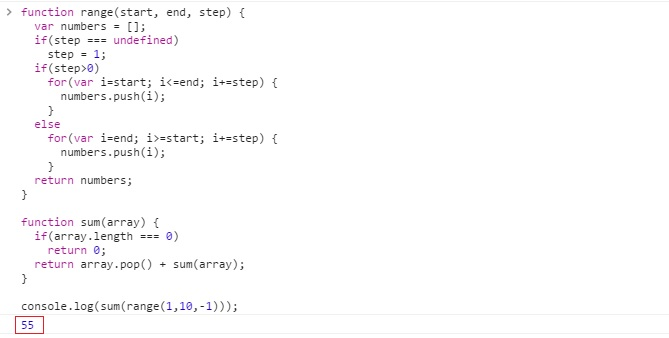# JavaScript program to print sum of range with steps

Learn: How to sum of the numbers through the range function in JavaScript? Range() takes two arguments start and end along with an optional argument step to determine step.
Submitted by Abhishek Pathak, on June 06, 2017

We are going to create a JavaScript program that has a range function which takes two arguments start and end along with an optional argument step to determine step. The use of this function is to return an array containing all the elements from start to end incrementing one by one if step is not defined.

Next, we are going to take a sum function that accepts array as argument and calculates the sum of the array elements. In this function, we will use recursion instead of for loop for the sake of sophistication in this simple program.

Also in the range function, if step is less than 1, i.e., -1, then range will be as follows [5,4,3,2,1]

## JavaScript code to find sum through range() function

```function range(start, end, step) {
var numbers = [];
if(step === undefined)
step = 1;
if(step>0)
for(var i=start; i<=end; i+=step) {
numbers.push(i);
}
else
for(var i=end; i>=start; i+=step) {
numbers.push(i);
}
return numbers;
}

function sum(array) {
if(array.length === 0)
return 0;
return array.pop() + sum(array);
}

console.log(sum(range(1,10,-1)));
```

Here is the output of JavaScript: